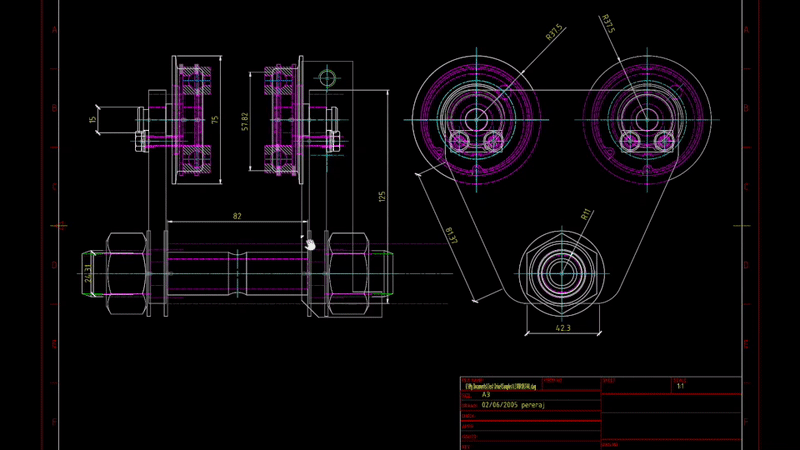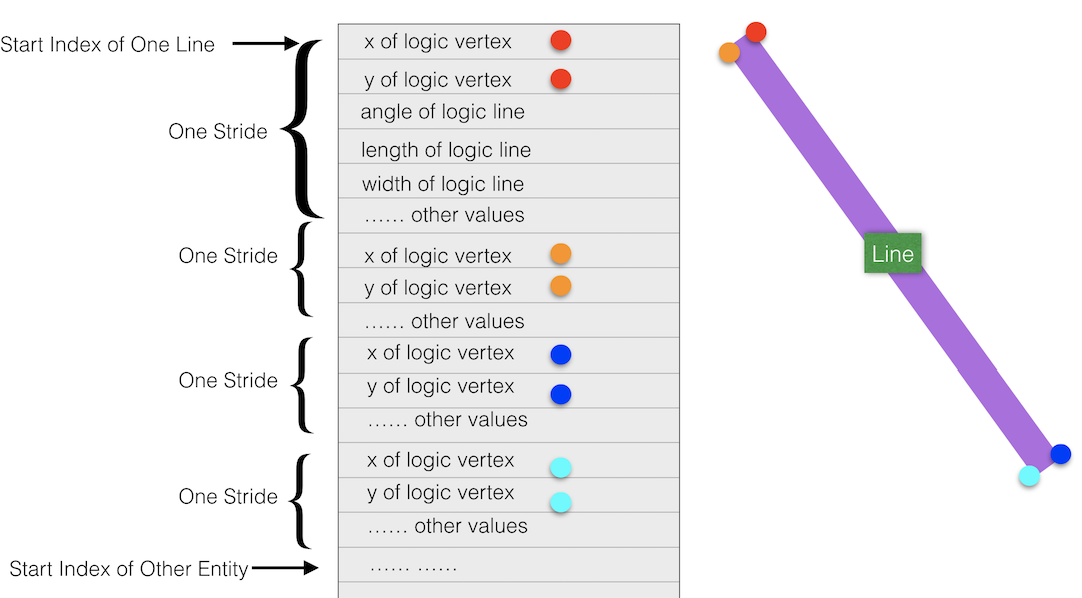July 7, 2019

# Move Entities of 2D DrawingNote:

• the code demo in this blog is NOT official methods. You would use at your own risk.

In the last blog, we introduced the way to get geometries of 2D curves by VertexBufferReader.enumGeomsForObject. A further question is asked frequently: how to move 2D entities? As mentioned in the last blog, it does not work if using the same workflow to manipulate 2D entities like 3D object. There is not either yet official method to transform 2D entities. We have logged the wish.

It is not easy unlike in 3D, while it is still possible to implement by ourselves with the clues of VertexBufferReader because the vertex values of the geometries is available. We could either try with

#1 hide the original geometries and create a new 2D vertex buffer at the new position.

#2 or modify the vertex buffer(s) in place to move the geometry for just the one object

For #1, we could simply reuse the code in the last blog, move the copied geometries, hide the original entities, but I have not figured out if it is a good idea to add copied geometries to the built-in vertex buffer. And it looks to me every time of moving will cause redundant data (hidden entities), unless we also take care of popping the original buffer .

For a line segment (on desktop machine) , there will be four vertices that make a quad which corresponds to the line segment. The code moves all of them.Triangles are encoded in three vertices (like a simple mesh) instead of 4 like everything else. It might be possible some triangles are connected in the buffer. They share some vertices. So we need to ensure transform the vertices one time only.Since VertexBufferReader tells the vertex buffer, it sounds more feasible with way #2. VertexBufferReader.enumGeomsForObject exposes the methods to get primitives of the geometries, unfortunately but they are cloned data from original vertex buffer. And it only tells the logic data, instead of full data that represents the geometry. In addition, RenderProxy.geometry.vb.buffer is the original data, but no method tells those vertices which belong to one entity (dbId).

So, I re-used the skeleton of VertexBufferReader.enumGeomsForObject. It iterates the buffer and filter out the start index of the vertices of specific entity (dbId). Next, some functions are created to transform the vertices. The code below is an extension to play with moving 2D entities. The live demo is at:

https://forge-viewer-unit-test.herokuapp.com/ent-2d/move.html

After model is loaded, click key ‘S’, select one curve, a cross indicator will shown up. Move mouse, the curve will be moved accordingly. Click ‘ESC’, you can select another curve and move. Click ‘Q’, the moving will be disable.

Note:

• the code demo in this blog is NOT official methods. You would use at your own risk.
• The code does not demo how to rotate, while it would be similar to moving.
• The code does not work if the entity has no fragments.
• The code only tries with Line/Arc/Circle/Triangles.
• The encoding of buffer is different depending on platform. The code demo is tested on desktop only.
``````//copy from VertexBufferReader.js
var VBB_GT_TRIANGLE_INDEXED = 0,
VBB_GT_LINE_SEGMENT     = 1,
VBB_GT_ARC_CIRCULAR     = 2,
VBB_GT_ARC_ELLIPTICAL   = 3,
VBB_GT_ONE_TRIANGLE     = 5;

var TAU = Math.PI * 2;
//end copy

if (this.useInstancing) {

////////
//TODO....
////////
}
else {

var i = 0;

//check the continuous triangles, to avoid double translating the same vertex of triangle
var ibArrayToMove =[];

while (i < this.ib.length) {
var vi = this.ib[i];
var flag = this.getVertexFlagsAt(vi);

//var vertexId    = (flag >>  0) & 0xff;        //  8 bit
var geomType    = (flag >>  8) & 0xff;        //  8 bit
//var linePattern = (flag >> 16) & 0xff;        //  8 bit
var layerId     = this.getLayerIndexAt(vi);    // 16 bit
var vpId        = this.getViewportIndexAt(vi); // 16 bit

var visible = this.getDbIdAt(vi) === dbId;

if (geomType === VBB_GT_TRIANGLE_INDEXED) {

//Triangles are encoded in three vertices (like a simple mesh) instead of 4 like everything else

if (visible) {
ibArrayToMove.push(this.ib[i]);
ibArrayToMove.push(this.ib[i+1]);
ibArrayToMove.push(this.ib[i+2]);

//do not translate at this moment because we have not yet known if the next primitive is
//one more triangle
}

i += 3;

} else {

if(ibArrayToMove.length>0){
//remove duplicated vertices
let unique_ibArrayToMove = [...new Set(ibArrayToMove)];
//translate all vertices now
this.transformTriangleIndexed(unique_ibArrayToMove, layerId, vpId, trans);

//reset the array for next continuous triangle
//good way to clear an array? no memory leak?
//see https://stackoverflow.com/questions/1232040/how-do-i-empty-an-array-in-javascript
ibArrayToMove=[];
}

if (visible) {

switch (geomType) {
case VBB_GT_LINE_SEGMENT:   this.transformLine( vi, layerId, vpId,trans);break;
case VBB_GT_ARC_CIRCULAR:   this.transformCircleArc(  vi, layerId, vpId, trans); break;
case VBB_GT_ARC_ELLIPTICAL:    //TODO break;
//case VBB_GT_ONE_TRIANGLE:        //TODO break;
default:                         break;
}
}

//Skip duplicate vertices (when not using instancing and the geometry is not a simple polytriangle,
//each vertex is listed four times with a different vertexId flag
i += 6;
}

}
}

//update the source buffer of the vertex
this.vb = this.vbf.buffer;
}

//custom function: transform vertex of a line
//layer, vpId: reserved arguments

var baseOffset = this.stride * vindex;

//For a line segment (on desktop machine)
//there will be four vertices that make a quad which corresponds to the line segment.

var i = 0;
while(i<4){
console.log('x:' + this.vbf[baseOffset + i*this.stride])
console.log('y:' + this.vbf[baseOffset + i*this.stride + 1])
this.vbf[baseOffset + i*this.stride] += trans.x;
this.vbf[baseOffset + i*this.stride + 1] += trans.y;
i++;
}
}

//custom function: transform vertex of a circle arc & circle
//layer, vpId: reserved arguments

var baseOffset = this.stride * vindex;

//arc or circle is also fit by line segments.
// For a line segment (on desktop machine)
//there will be four vertices that make a quad which corresponds to the line segment.
var i = 0;
while(i<4){
this.vbf[baseOffset + i*this.stride] += trans.x;
this.vbf[baseOffset + i*this.stride + 1] += trans.y;
i++;
}
}

//custom function: transform vertex of triangles
//layer, vpId: reserved arguments
function(ibArray, layer, vpId, trans)
{
var k = 0;
while(k<ibArray.length){
var baseOffset = this.stride * ibArray[k];
this.vbf[baseOffset] += trans.x;
this.vbf[baseOffset + 1] += trans.y;
k++;
}

};

function My2DMove(viewer, options) {

Autodesk.Viewing.Extension.call(this, viewer, options)

var _viewer = this.viewer
var _container = _viewer.canvas.parentElement

var indicatorLines = null
var indicatorLayerName = 'indicatorLayer'
var indicatorLineBias = 0.2
//is mouse moving
var _dragging = false
//selected object id
var _selectDbId = 0

//current point of when mouse is moving
var _mouseStPt = new THREE.Vector3(0,0,0);
//last mouse point
var _mouseLastPt = new THREE.Vector3(0,0,0);

//bind keyup event
\$(document).bind('keydown', onKeyUp);
_viewer.impl.invalidate(true);
return true;
};

//unbind keyup event
\$(document).unbind('keyup', this.onKeyUp);
return true;
};

//when key up
function onKeyUp(evt) {
console.log('onKeyUp:' + evt.keyCode);

//when key 'S' is pressed
if(evt.keyCode == 83){
//start to monitor mouse down
}

//when key 'Q' is pressed
if(evt.keyCode == 81){
_viewer.removeEventListener(Autodesk.Viewing.SELECTION_CHANGED_EVENT, onSelectObj)

//remove mouse events
_container.removeEventListener('click',onMouseClick);
_container.removeEventListener('mousemove',onMouseMove);

_viewer.impl.removeOverlayScene(indicatorLayerName);
_viewer.impl.invalidate(false, false, true);
_dragging = false
}

return true;
}

function onSelectObj(evt){
console.log(evt)
_selectDbId = evt.dbIdArray
}

function onMouseClick(evt) {
if(!_dragging){
var pt_3d = _viewer.clientToWorld(evt.clientX,evt.clientY)
createIndicator(pt_3d.point)
_dragging = true
}
}

function onMouseMove(evt) {
if(_dragging){
//get mouse points
var pt_3d = _viewer.clientToWorld(evt.clientX,evt.clientY)
moveIndicator(pt_3d.point)
}
}

function createIndicator(pt){

var geometry = new THREE.Geometry ()
geometry.vertices.push (new THREE.Vector3 ( pt.x-indicatorLineBias,  pt.y,  0))
geometry.vertices.push (new THREE.Vector3 ( pt.x+indicatorLineBias, pt.y, 0))
geometry.vertices.push (new THREE.Vector3 ( pt.x, pt.y-indicatorLineBias, 0))
geometry.vertices.push (new THREE.Vector3 ( pt.x, pt.y+indicatorLineBias, 0))

var linesMaterial = new THREE.LineBasicMaterial ({
color: new THREE.Color (0xFF0000),
transparent: true,
depthWrite: false,
depthTest: false,
linewidth: 3,
opacity: 1.0
})

indicatorLines = new THREE.Line (geometry,
linesMaterial,
THREE.LinePieces)

_viewer.impl.createOverlayScene (indicatorLayerName, linesMaterial)
_viewer.impl.invalidate (false,false,true)

_mouseLastPt.x = _mouseStPt.x = pt.x
_mouseLastPt.y = _mouseStPt.y = pt.y
}

function moveIndicator(pt){

if(indicatorLines != null){

var diffX = pt.x - _mouseStPt.x
var diffY = pt.y - _mouseStPt.y

indicatorLines.position.x = diffX
indicatorLines.position.y = diffY

_viewer.impl.invalidate(false, false, true)

move2DEnt = move2DEnt(_selectDbId,{x:pt.x - _mouseLastPt.x,y:pt.y - _mouseLastPt.y })
_mouseLastPt = pt
}

function move2DEnt(dbId,trans){
var it = _viewer.model.getData().instanceTree;
it.enumNodeFragments( dbId, function( fragId ) {
var m = _viewer.impl.getRenderProxy(_viewer.model, fragId);
_viewer.impl.use2dInstancing);
vbr.transformObject(dbId,trans);
m.geometry.vbNeedsUpdate = true;
_viewer.impl.sceneUpdated()
});
}

}
}

My2DMove.prototype = Object.create(Autodesk.Viewing.Extension.prototype);
My2DMove.prototype.varructor = My2DMove;

Autodesk.Viewing.theExtensionManager.registerExtension('My2DMove', My2DMove);
``````